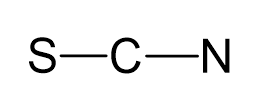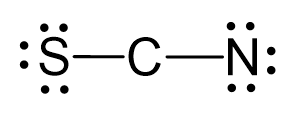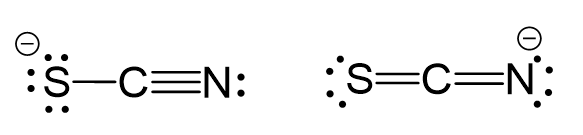## Examples

Carbon is the central atom:There are 6 + 4 + 5 + 1 = 16 electrons, and 4 are already taken to make two covalent bonds. The remaining 12 are divided between the S and N as 3 lone pairs:One possibility is to use two lone pairs from the nitrogen, and the other is to once form the nitrogen and one form the sulfur:These two are resonance forms of the same compound either one gives a linear electron molecular geometry because the central atom has two atoms and no lone pairs.

Steric number 2 corresponds to sp-hybridization where the bond angles are 180o